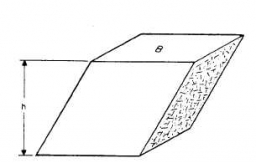The base of the quadrilateral prism is a diamond, which has diagonals of 7 and 9 cm. The height of the prism is 22 cm. What is the area?

S =  564.6772 cm2

### Step-by-step explanation:Did you find an error or inaccuracy? Feel free to write us. Thank you!

Tips for related online calculators
The Pythagorean theorem is the base for the right triangle calculator.

#### You need to know the following knowledge to solve this word math problem:

We encourage you to watch this tutorial video on this math problem: